# Precalculus : Determine the Polar Equation of a Graph

## Example Questions

### Example Question #1 : Graphs Of Polar Equations

Which polar equation would produce this graph?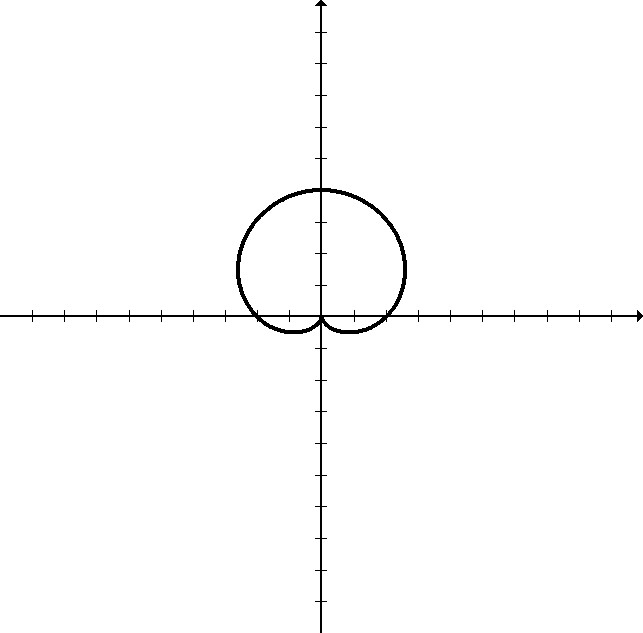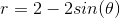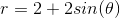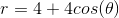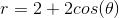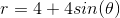Explanation:

This is the graph of a cardiod. Based on its orientation where the cusp [pointy part] is on the y-axis, it is a sine and not cosine function. The x-intercepts are at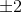, so the first number must be 2. Since vertically the graph goes from 0 to 4, the second number must be 2, because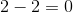and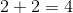.

### Example Question #1 : Graphs Of Polar Equations

Give the polar equation for this graph: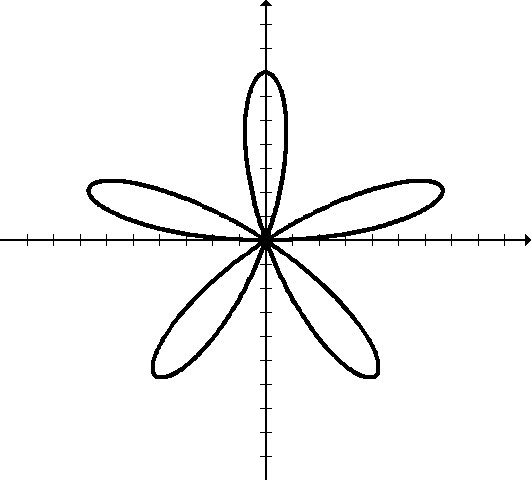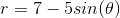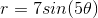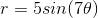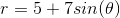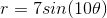Explanation:

This graph shows a rose curve with an odd number of petals.

This means that the equation will be in the form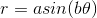, whererepresents the length of the "petals" andrepresents the number of petals.

There are 5 petals of length 7.

### Example Question #1 : Determine The Polar Equation Of A Graph

Which polar equation would produce this graph?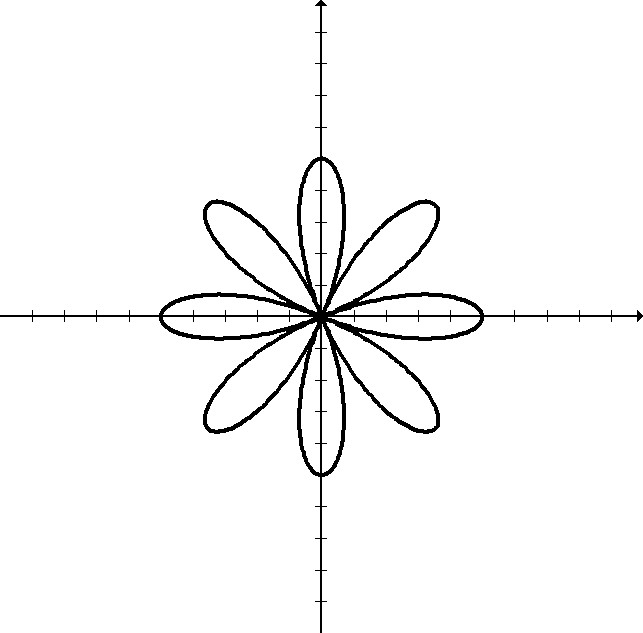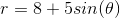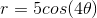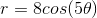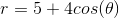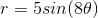Explanation:

This graph shows a rose curve with an even number of petals. The first petal also intersects with the x-axis. This means that the equation will be in the form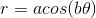whereis the length of each petal, andis half the number of petals. (Note that for an odd number of petals, the rose curve will have exactlypetals). In this case, the petals have length 5, and there are 8 of them [half of 8 is 4].

### Example Question #4 : Graphs Of Polar Equations

Determine the equation of the following polar graph: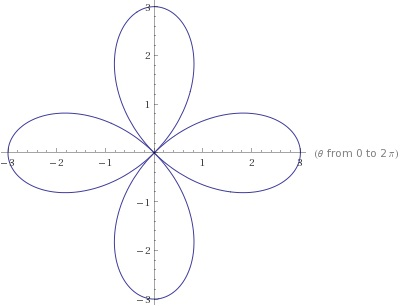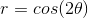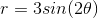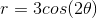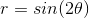Explanation:

Each loop is on the line of each axis, which means the equation will have a cosine.

The loops stretch out to the third unit on each axis, which means there will be a three in front of the cosine.

Lastly, there are 4 loops. Whenever there is an even number of loops, you divide that number by 2, and that is the number which goes in front of theta in the parentheses. In this case, it is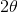because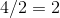..